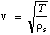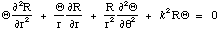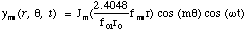Swarthmore College

Engineering Methodology

In lecture this week, you learned about vibrations of solids and membranes. The Matlab assignment below asks you to plot in 3-D and animate the motion of a circular drumhead, based upon equations for the radial and circumferential nodal patterns..

Problem

A circular drumhead can vibrate in any way that satisfies both the boundary conditions and the wave equation for flexural waves in an elastic membrane. If y is the vertical deflection, r and q are the radial and circumferential coordinates of each point on the circular membrane, and t is the time coordinate, then the wave equation can be written:, where, (1)

is the wave speed,T is the tension, and rs is the mass per unit area of the membrane material. If we assume a solution of the form y(r, q, t) = R(r) Q(q) ejwt, where each of the components is dependent on only one of the dimensions as indicated, Equation 1 can be written as:where k = w/v. (2)

Equation 2 is in the form of a Helmholtz equation. Collecting all the terms containing r on one side of the equation and those containing q on the other, we make the observation that the two sides can equal each other for all times t only if they equal a constant, which we will call m2. The Q-side of the equation becomes a differential equation with solutions Q(q) = cos (mq + g), where if displacement y is to be a single-valued function of position, m must bem = 0, 1, 2, 3, ... . The R-side of the equation becomes Bessel's equation, whose solutions R(r) are transcendental functions called Bessel functions of the first kind, Jm(kr), and of the second kind, Ym(kr), both which you can find tabulated (like trig functions):

R(r) = A Jm(kr) + B Ym(kr) (3)

In Matlab, the function besselj(m,k*r) gives Jm(kr) and the function bessely(m,k*r) gives Ym(kr) as you can plot for yourself using the following commands:

x = linspace(0,10,100);

jbess = besselj(0,x);

ybess = bessely(0,x);

plot(x,jbess,x,ybess);

These solutions to Equation 2, R(r) and Q(q), are general and require the imposition of boundary conditions to limit the allowed frequencies to a discrete set. Since the Ym(kr) functions go to negative infinity at the origin, B must equal zero (this would not be the case for an annular membrane). Likewise since the membrane is fixed at the rim, R(r=r0) = 0 so

Jm(kr0) = 0. If the values of the argument of the mth order Bessel function Jm that cause that function to equal zero are designated by jmn, then the corresponding values of k assume discrete values given by kmn = jmn/r0. The physical motion of the normal modes designated by the integer pairs (m, n) is therefore:, (4)

where j01 = 2.4048 has been included and the first few of the frequencies fmn expressed relative to the fundamental frequency f01 are as follows:

 f01 = 1.0 f01 f11 = 1.593 f01 f21 = 2.135 f01 f02 = 2.295 f01 f12 = 2.917 f01 f22 = 3.500 f01 f03 = 3.598 f01 f13 = 4.230 f01 f23 = 4.832 f01

The patterns of vibration consist therefore of independent combinations of nodal lines (the number of which is given by the first subscript m = 0, 1, 2, 3,...) and nodal circles (given by n = 1, 2, 3,... since the rim counts as the first nodal circle), in keeping with whatever additional imposed boundary conditions exist at the instant of excitation (for instance, the strike point must be an antinode, and a finger placed on the drumhead forces a node there).

Write a Matlab script that prompts the user for m and n and displays the membrane shape as a function of time in three-dimensional graphics. "Animate" the motion by repeated plotting at different snapshots during one period of vibration. Using the drop box on the Classes file server, hand in your script only (with your username followed by the number 8 as the script name before the ".m").

Solution

Begin by creating, via the Matlab™ command window, a variable fmn defined as the matrix of the frequency coefficients in the table above (use semicolons at the end of each row, commas between). Save the contents of the variable in a .mat file using the save command. Your Matlab script should begin by opening and reading in the data from the file (use the load command), then prompt the user for the number of nodal lines m and the number of nodal circles n to be displayed. By using subscripts, verify that you can pick out the correct frequency coefficient from the matrix (f = fmn(n,m+1) works nicely, but verify this).

Ask the user for the radius of the drumhead and store it in the variable radius. Next, create a variable resol to hold the resolution of the grid that will specify the surface (50 points, for example) and use this number in the linspace command to create the x and y arrays and the X and Y matrices of the domain (use the meshgrid command; see Section 5.20.2, p. 169-178 in your book). As p. 170 shows, all that is left is to specify the function of x and y to be plotted and call the mesh command.

To plot an x-y surface using r and q, define R as on p. 170 and theta using the atan2 function to preserve the correct sign in quadrants II and III (you can look up this function in the index and read about it. Essentially you will use the command theta = atan2(Y,X)). We would like to restrict the surface oscillations to those within the circular domain R ˛ radius, so create a matrix rim of the same size as R but with the radius at every position (rim = radius*ones(resol) will work; it uses the ones command to generate a matrix of ones). The logical expression (R<=rim) is evaluated as a 1 ('true') whenever the value of the matrix R is less than or equal to the radius number in rim, or a 0 ('false') when the value is outside it. Use this expression to multiply any function (see p. 82-83) you wish to be plotted only within the circular domain R ˛ radius.

The function to plot Equation 4 will make use of the besselj function (see p. 331) of order m times the cosine of m*theta. Remembering that we wish to do an element-by-element multiplication (.*), the line will look something like this:

Z = (R <= rim).* besselj(m,f*2.4048/radius*R).*cos(m*theta);

Leave off the cos(wt) part for the evaluation of the surface in the line above, but then put in a loop that multiplies the surface Z by the cos(wt) term (which will range between 1 and -1). The loop will look something like this, if you normalize the vertical z-axis by the highest point in the surface matrix Z:

tall = max(max(Z)); % save biggest number in matrix for scaling

for i=1:11 % loop eleven time increments per cycle

newZ = Z*cos(i*2*pi/11);

mesh(newZ);

axis([0, resol, 0, resol, -tall, tall]);

pause(0.5);

end

Challenge assignment (only if you find the assignment above unchallenging)

Make the above program into a real animation using Matlab's moviein and movie commands.

Matlab solution